# What is JupyterLab?

`C:\Users\Janvi>jupyter lab`

# Prerequisites

`pip install librosa soundfile numpy sklearn pyaudio`

# Steps for speech emotion recognition python projects

`import librosaimport soundfileimport os, glob, pickleimport numpy as npfrom sklearn.model_selection import train_test_splitfrom sklearn.neural_network import MLPClassifierfrom sklearn.metrics import accuracy_score`
• mfcc: Mel Frequency Cepstral Coefficient, represents the short-term power spectrum of a sound
• chroma: Pertains to the 12 different pitch classes
• mel: Mel Spectrogram Frequency
`def extract_feature(file_name, mfcc, chroma, mel):   with soundfile.SoundFile(file_name) as sound_file:   X = sound_file.read(dtype="float32")   sample_rate=sound_file.samplerate   if chroma:   stft=np.abs(librosa.stft(X))   result=np.array([])   if mfcc:   mfccs=np.mean(librosa.feature.mfcc(y=X,sr=sample_rate,   n_mfcc=40).T,axis=0)   result=np.hstack((result, mfccs))   if chroma:   chroma=np.mean(librosa.feature.chroma_stft(S=stft,    sr=sample_rate).T,axis=0)   result=np.hstack((result, chroma))   if mel:   mel=np.mean(librosa.feature.melspectrogram(X,  sr=sample_rate).T,axis=0)   result=np.hstack((result, mel))return result`
`emotions={'01':'neutral','02':'calm','03':'happy','04':'sad','05':'angry','06':'fearful','07':'disgust','08':'surprised'}observed_emotions=['calm', 'happy', 'fearful', 'disgust']`
`def load_data(test_size=0.2):   x,y=[],[]   for file in glob.glob("/content/sample_data/mini project/*.wav"):      file_name=os.path.basename(file)      emotion=emotions[file_name.split("-")]      if emotion not in observed_emotions:         continue      feature=extract_feature(file, mfcc=True, chroma=True, mel=True)      x.append(feature)      y.append(emotion)      return train_test_split(np.array(x), y, test_size=test_size,  random_state=9)`
`x_train,x_test,y_train,y_test=load_data(test_size=0.15)`
`print((x_train.shape, x_test.shape))`
`print(f'Features extracted: {x_train.shape}')`
`model=MLPClassifier(alpha=0.01, batch_size=256, epsilon=1e-08, hidden_layer_sizes=(300,), learning_rate='adaptive', max_iter=500)`
`model.fit(x_train,y_train)`
`y_pred=model.predict(x_test)`
`accuracy=accuracy_score(y_true=y_test, y_pred=y_pred)print("Accuracy: {:.2f}%".format(accuracy*100))`

# Summary

--

--

--

## More from Janvi Ajudiya

2x AWS Certified

Love podcasts or audiobooks? Learn on the go with our new app.

## Let me tell you a lie: it’s average## 6 Tips to Make Data Visualization More Effective## Which Curve To Follow? Using Precedent Outlook Scenarios to Inform Local Planning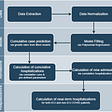## Control your Airflow DAG from an external database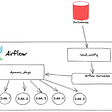## Unlocking our data with a feature store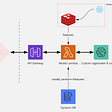## Rush Limbaugh is the smartest man in America?## How Data Science & Machine Learning are Revolutionizing The Film Industry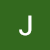2x AWS Certified

## Machine Learning is Fun! (part 2)## A New Proteomics Dataset Search Engine## Diabetes Prediction Web App!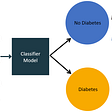## Applied ML End to End Journey — Step 2, Part 1